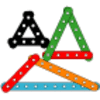#### You may also like### I'm Eight

Find a great variety of ways of asking questions which make 8.### Let's Investigate Triangles

Vincent and Tara are making triangles with the class construction set. They have a pile of strips of different lengths. How many different triangles can they make?### Noah

Noah saw 12 legs walk by into the Ark. How many creatures did he see?

# Are You Well Balanced?

## Are You Well Balanced?

You might like to have a look at Number Balance before trying this problem.

Here is a number balance, or equaliser:

Full Screen Version

It has weights hanging off it to make it balance.
We can hang more than one weight from each hook.
I hang one weight on the $10$. I want to use just one hook on the other side of the equaliser.
Where could I put weights and how many would I need to put on?
Now I hang two weights on the $10$ on one side of the equaliser.
Using only the $2$ hook, how could I add weights on the other side to make it balance?
Using only the $5$ hook, how could I add weights on the other side to make it balance?
How could you make it balance using both the $2$ and the $5$ hooks? Is there only one solution?
Now I hang one weight on the $10$ hook on one side of the equaliser.
If I use the $3$ hook and one other, how can I make it balance?

### Why do this problem?

This problem can be used to introduce repeated addition and therefore concepts of multiplication. It could also be used to challenge children to work systematically to find all possible solutions.

### Possible approach

Before tackling this problem, pupils will need practical experience of working with balances, if possible. It would also be a good idea to have a look at Number Balance which uses the same interactivity, but focuses on number bonds.

To introduce the problem, show the class the balance on the interactive whiteboard and, without saying anything, hang weights to make it balance, perhaps one on the left and two on the right. Take the weights off and put on a different combination of weights on each side to balance the equaliser. Repeat this a few times and invite learners to talk to each other in pairs about what they think is happening. You could then try the first question as a whole group, asking the children to talk to each other about what to do before sharing ideas and checking using the interactivity. During this discussion, you can specifically introduce language to help children talk about what they are doing, for example "$2$ add $2$ add $2$ add $2$ add $2$ equals $10$ or five lots of $2$ equal $10$".

For the other parts of the problem, you may want learners to be at a computer in pairs or you may prefer them to work on mini-whiteboards/paper. After leaving time for them to work on this, bring them together and discuss their solutions. When it comes to considering balancing a total of $20$ on one side with weights on both the $2$ hook and the $5$ hook, you may want to ask pairs to write up some solutions on individual strips of paper which can be stuck on the board. You could look for patterns in the solutions and order them to help decide whether any have been missed out. Having seen this, children could try to use a similar system to find all the solutions to the final part of the problem.

### Key questions

Where have you tried hanging weights so far?
How will you know that you have got all the ways of making the equaliser balanced?

### Possible extension

Children could investigate ways of balancing the equaliser if, for example, you can only put weights on the $2$ hook on one side and the $10$ hook on the other.

### Possible support

Having access to the interactivity, either on the whiteboard, or on individual computers, will help some children gain in confidence as they will be able to try out their ideas without the anxiety of getting things wrong.